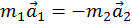Remove from favorites

# Newton's third law of motion calculator

Newtons third law of motion calculator allows you to calculate a mass and acceleration of two objects by using newtons third law of motion.

## Calculate by the Newton's third law

Acceleration (a1): m/s2
Mass (m2): kg
Acceleration (a2): m/s2Newton's third law of motion calculator states that all forces between two objects exist in equal magnitude and opposite direction.